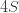# The Sum of the Natural Numbers is -1/12?

Posted on the 10 February 2014 by Ccc1685 @ccc1685

This wonderfully entertaining video giving a proof for why the sum of the natural numbers  is -1/12 has been viewed over 1.5 million times.  It just shows that there is a hunger for interesting and well explained math and science content out there.   Now, we all know that the sum of all the natural numbers is infinite but the beauty (insidiousness) of infinite numbers is that they can be assigned to virtually anything.  The proof for this particular assignment considers the subtraction of the divergent oscillating sumfrom the divergent sum of the natural numbersto obtain.  Then by similar trickery it assigns.  Solving forgives you the result.  Hence, what you are essentially doing is dividing infinity by infinity and that as any school child should know can be anything you want.  The most astounding thing to be about the video was learning that this assignment was used in string theory, which makes me wonder if the calculations would differ if I chose a different assignment.

See more